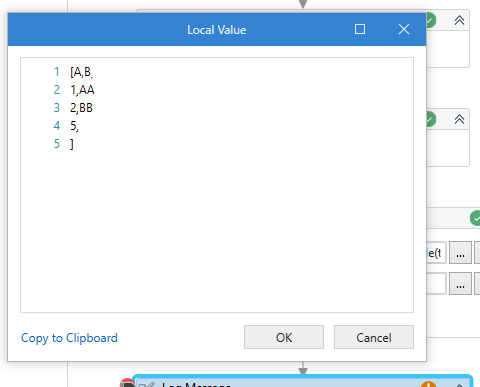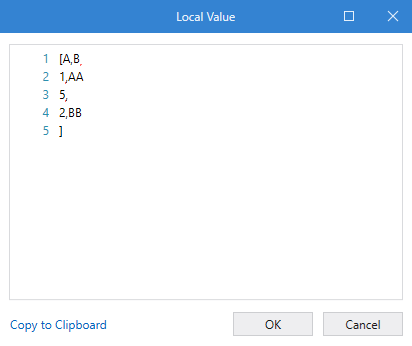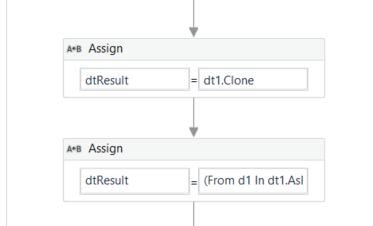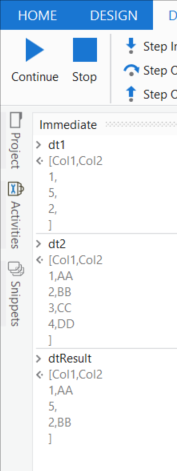# Get value to DT from other DT using Linq

Hi everyone,
Now I working on excel with bunch of row, I try to get value from DT_Master, to DT_Input, when value from DT_Input is match with value in DT_Master. For example
DT_Input
A B
1
5
2

DT_Master
A B
1 AA
2 BB
3 CC
4 DD

Final result that I want is
DT_Input
A B
1 AA
5
2 BB
(While it’s still maintains the position of the results as before, 5 still in row number 2)

Now I’m using for each row in for each row and takes 2 hours to complete the task, I also try using linq but I stuck with this code
(From DT1 In DT_Master.AsEnumerable
Let fltr = DT_Input.AsEnumerable.Any(Function (DT2) DT2(“Number”).toString.Equals(DT1(“Number”).toString.Trim))
Where fltr
Select r=DT1).CopyToDataTable

Thanks for reply and have a nice day!

Join Data Tables will help with `Left Join`

Here is the sample,Hope this helps.

Thanks
#nK

1 Like

You can use a join table activity. Join statements are really fast comapred to both linq and nested for loops.
In join table activity, use a left join and put dt_input in datatable 1 and put dt_Master in datatable 2.
if you want you can use a filter table and output the required columns

1 Like

see an example here to join tables using LINQ

1 Like

Hai, thank you for the reply!

I used Left Join before, but I need the row position of the DT_Input is same as before, because I just want to write the value from DT_Master to DT_Input, if I use Left Join it will join the same value then write the rest after. For example
DT_Input
A B
1
5
2

DT_Master
A B
1 AA
2 BB
3 CC
4 DD

Result if I use Left Join
A B
1 AA
2 BB
5

Result I want
A B
1 AA
5
2 BB
(5 is still in the position of row number 2)

I used Left Join before, but I need the row position of the DT_Input is same as before, because I just want to write the value from DT_Master to DT_Input, if I use Left Join it will join the same value then write the rest after. For example
DT_Input
A B
1
5
2

DT_Master
A B
1 AA
2 BB
3 CC
4 DD

Result if I use Left Join
A B
1 AA
2 BB
5

Result I want
A B
1 AA
5
2 BB
(5 is still in the position of row number 2)
Sorry I copied the reply above, because the solution you gave is the same.

I used Left Join before, but I need the row position of the DT_Input is same as before, because I just want to write the value from DT_Master to DT_Input, if I use Left Join it will join the same value then write the rest after. For example
DT_Input
A B
1
5
2

DT_Master
A B
1 AA
2 BB
3 CC
4 DD

Result if I use Left Join
A B
1 AA
2 BB
5

Result I want
A B
1 AA
5
2 BB
(5 is still in the position of row number 2)
Sorry I copied the reply above, because the solution you gave is the same

Okay clear.

Kindly check this flow.Thanks
#nK

1 Like

Thanks a lot! I have try it on my flow, and its works perfectly! But if you don’t mind, can you give me a fast explanation about what are you doing in the second assign? I try to understand but don’t get it clearly

1 Like

Here it is,

``````//Select is always to customize the view of the DT
DT_Input.AsEnumerable.Select(Function(row)

//DT Rows add function to create the new rows with matching values

//Item Array of Objects formation to add as a row
{ row("A").ToString.Trim,  //First DT first column added as it is

//For second column, we are trying to match and find from 2nd DT, if not adding empty string
If(DT_Master.AsEnumerable.Any(Function(row2) row2("A").ToString.Trim.Equals(row("A").ToString.Trim)), DT_Master.AsEnumerable.First(Function(row2) row2("A").ToString.Trim.Equals(row("A").ToString.Trim)).Item("B").ToString.Trim, String.Empty)}
)).CopyToDataTable    //Finally copying to DT
``````

I’m not sure if I explained it easily or a bit complicated wayBut if you didn’t get it please let me know.

Thanks
#nK

1 Like

woahh, thank you very much, it’s all clear now, have a nice day!1 Like

@Hendaryie_Tjoeng
Left Join LINQ respecting the ordering would look like this:``````(From d1 In dt1.AsEnumerable
Group Join d2 In dt2.AsEnumerable
On d1(0).toString.ToUpper.Trim Equals d2(0).toString.ToUpper.Trim Into gj = Group
From j In gj.DefaultIfEmpty()
Let b = If(isNothing(j), Nothing, j(1))
Order By dt1.Rows.IndexOf(d1)
Let ra = New Object(){d1(0), b}
``````Kindly note: maybe an optimzation on Dictionary base is to check when execution requires some tuning:

1 Like

for the Order By line, its means you can use index of DT_Input to the DT_Result just like so?

With the let statement we can memorize for later usage
Here we check if there is right side join pair then use the col2 value otherwise it is set to null
later when constructing the row itemArray we use the prepared value - b

Yes we order the LINQ result on the left side datatable rows as mentioned by you about the requirements

1 Like

thank you very much! and thanks for linq learning post, it really helps to understand linq, have a nice day1 Like

This topic was automatically closed 3 days after the last reply. New replies are no longer allowed.